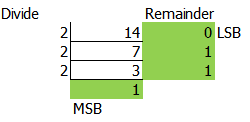How To Convert Base number

On this article I will show how to convert from Number base 10 system to other base system.

Convert Number Base 10 (Decimal) to Base 2

• Convert 14  to base 214 will equal to 11102

Convert Number Base 2 to Base 10(Decimal)

• Convert 11102 to Decimal14 = 8 + 4 + 2 + 0

11102 = 14

Convert Number Base 10 (Decimal) to Base 16(Hex)

• Convert 256 to base 16256 = 10016

Convert Number Base 2 to Base 10(Decimal)

• 10016   to Decimal256 = 256 + 0 + 0

10016 = 256